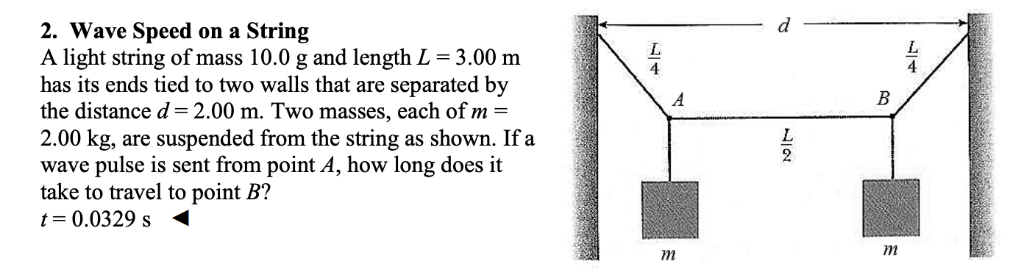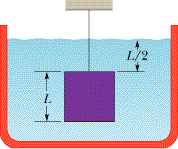Ask question

# Rhombus IKLM has vertices J(−1,−4), K(1,1), and L(6,3). The coordinates of M are (□)⋅(). [17,6]5.D# Rhombus IKLM has vertices J(−1,−4), K(1,1), and L(6,3). The coordinates of M are (□)⋅(). [17,6]5.D

Question
Solid Geometryasked 2020-12-07
Rhombus IKLM has vertices J(−1,−4), K(1,1), and L(6,3). The coordinates of M are (□)⋅(). [17,6]5.D

## Answers (1)2020-12-08
A rhombus is a parallelogram so its opposite sides are parallel. So, JK¯¯¯¯¯∥LM¯¯¯¯¯¯. To get from K to J, you move 2 units to the left (subtract 2 from x) and move 5 units down (subtract 5 from y). So, we to this from L to find M:
M(6−2,3−5)=M(4,−2)

### Relevant Questionsasked 2021-05-06
The coefficient of linear expansion of copper is 17 x 10-6 K-1. A sheet of copper has a round hole with a radius of 3.0 m cut out of it. If the sheet is heated and undergoes a change in temperature of 80 K, what is the change in the radius of the hole? It decreases by 4.1 mm. It increases by 4.1 mm. It decreases by 8.2 mm. It increases by 8.2 mm. It does not change.asked 2021-04-30
Two oppositely charged but otherwise identical conducting plates of area 2.50 square centimeters are separated by a dielectric 1.80 millimeters thick, with a dielectric constant of K=3.60. The resultant electric field in the dielectric is $$\displaystyle{1.20}\times{10}^{{6}}$$ volts per meter.
Compute the magnitude of the charge per unit area $$\displaystyle\sigma$$ on the conducting plate.
$$\displaystyle\sigma={\frac{{{c}}}{{{m}^{{2}}}}}$$
Compute the magnitude of the charge per unit area $$\displaystyle\sigma_{{1}}$$ on the surfaces of the dielectric.
$$\displaystyle\sigma_{{1}}={\frac{{{c}}}{{{m}^{{2}}}}}$$
Find the total electric-field energy U stored in the capacitor.
u=Jasked 2021-02-16
Three building materials, plasterboard [k = 0.30 J/(s m Co)], brick [k = 0.60 J/(s m Co)], and wood [k = 0.10 J/(s m Co)], are sandwiched together as the drawing illustrates. The temperatures at the inside and outside surfaces are $$\displaystyle{25.1}^{\circ}$$ C and $$\displaystyle{0}^{\circ}$$ C, respectively. Each material has the same thickness and cross-sectional area. Find the temperature (a) at the plasterboard-brick interface and (b) at the brick-wood interface.asked 2021-04-20
d 2. Wave Speed on a String A light string of mass 10.0 g and length L = 3.00 m has its ends tied to two walls that are separated by the distance d=2.00 m. Tasked 2021-02-01
Three vertices of parallelogram DEFG are D(-2, -4), F(0, 7), and G(1, 0). Find the coordinates of E. The coordinates of E are ___.asked 2021-02-23
A 0.30 kg ladle sliding on a horizontal frictionless surface isattached to one end of a horizontal spring (k = 500 N/m) whoseother end is fixed. The ladle has a kinetic energy of 10 J as itpasses through its equilibrium position (the point at which thespring force is zero).
(a) At what rate is the spring doing work on the ladle as the ladlepasses through its equilibrium position?
(b) At what rate is the spring doing work on the ladle when thespring is compressed 0.10 m and the ladle is moving away from theequilibrium position?asked 2021-04-25
The unstable nucleus uranium-236 can be regarded as auniformly charged sphere of charge Q=+92e and radius $$\displaystyle{R}={7.4}\times{10}^{{-{15}}}$$ m. In nuclear fission, this can divide into twosmaller nuclei, each of 1/2 the charge and 1/2 the voume of theoriginal uranium-236 nucleus. This is one of the reactionsthat occurred n the nuclear weapon that exploded over Hiroshima, Japan in August 1945.
A. Find the radii of the two "daughter" nuclei of charge+46e.
B. In a simple model for the fission process, immediatelyafter the uranium-236 nucleus has undergone fission the "daughter"nuclei are at rest and just touching. Calculate the kineticenergy that each of the "daughter" nuclei will have when they arevery far apart.
C. In this model the sum of the kinetic energies of the two"daughter" nuclei is the energy released by the fission of oneuranium-236 nucleus. Calculate the energy released by thefission of 10.0 kg of uranium-236. The atomic mass ofuranium-236 is 236 u, where 1 u = 1 atomic mass unit $$\displaystyle={1.66}\times{10}^{{-{27}}}$$ kg. Express your answer both in joules and in kilotonsof TNT (1 kiloton of TNT releases 4.18 x 10^12 J when itexplodes).asked 2021-03-24
A 2.4-kg object is attached to a horizontal spring of forceconstant k=4.5 kN/m. The spring is stretched 10 cm fromequilibrium and released. Find (a) the frequency of themotion, (b) the period, (c) the amplitude, (d) the maximum speed,and (e) the maximum acceleration. (f) When does the objectfirst reach its equilibrium position? What is itsacceleration at this time?
Two identical blocks placed one on top of the other rest on africtionless horizontal air track. The lower block isattached to a spring of spring constant k= 600 N/m. Whendisplaced slightly from its equilibrium position, the systemoscillates with a frequency of 1.8 Hz. When the amplitude ofoscillation exceeds 5 cm, the upper block starts to slide relativeto the lower one. (a) What are the masses of the twoblocks? (b) What is the coefficient of static frictionbetween the two blocks?asked 2021-04-19
In the figure, a cube of edge length L = 0.599 m and mass 970 kg is suspended by a rope in an open tank of liquid of density 1.05E+3 kg/m3. Find (a) the magnitude of the total downward force on the top of the cube from the liquid and the atmosphere, assuming atmospheric pressure is 1.00 atm, (b) the magnitude of the total upward force on the bottom of the cube, and (c) the tension in the rope. (d) Calculate the magnitude of the buoyant force on the cube using Archimede's principle.asked 2021-02-23
An electron that has velocity $$\displaystyle{v}={\left({2.0}\times{10}^{{6}}\ \frac{{m}}{{s}}\right)}{i}+{\left({3.0}\times{10}^{{6}}\ \frac{{m}}{{s}}\right)}{j}$$ moves through the uniform magneticfield $$\displaystyle{B}={\left({0.30}{T}\right)}{i}-{\left({0.15}{T}\right)}{j}$$
a) Find the force on the electron.
b) Repeat your calculation for a proton having the same velocity.
...